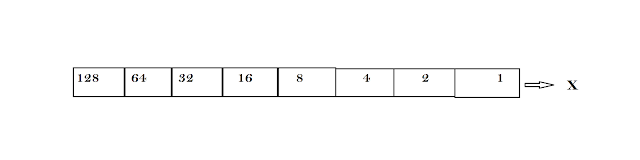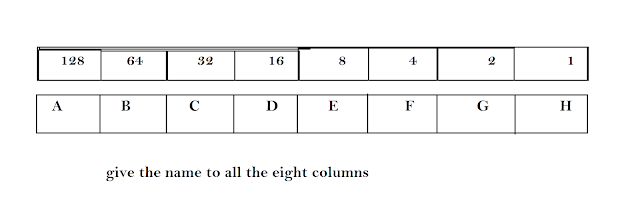# Count binary numbers and range of operating system

Count binary numbers: In this post, you will learn about how to count binary numbers and how to find the range of different types of operating systems.Binary Number

## Binary Numbers:

The binary number is a combination of 0 and 1.
Note: if you want to know in detail that what is binary number and how it works in the computer system. then you should need to read this post.

First of all, you need to know that we also called 0 and 1 to an off or on. So how do a 125 number representing in base two numbers in the bit system?

How we calculate it. so let find it.

First of all, we need to know how many bits we working with. and how is our system? like 8,16,32 and 64 bit system.

let's take an example we work with the 8-bit system.
so, first of all, we need to draw 8 columns. and by simple addition, we get binary data.
lets I explain step by step

### How to find the range of the operating system?

STEP 1
Draw the boxes with the help of your system. like draw 8 columns in the 8-bit system and draw 64 columns in a 64-bit system.

STEP 2
Store with the least significant bits on the right-hand side. and in every column, we need to double up our number. like 1 and next 2, next 4, next 8, next 16, next 32, next 64, and soon. and also give the name to the columns as shown in figure 2.fig. 2
so lets we get the range of numbers. to know the range of numbers follows the steps given below.

STEP 3

First of all, add all the numbers given in the column.
1+2+4+8+16+32+64+128=255

and put all the numbers into the columns equal to the zero as shown in fig3.fig. 3

0+0+0+0+0+0+0+0=0

so we get the range of 8 bit systems. which is 0 to 255.
so we can generate all numbers between 0 to 255 and converted into 2-bit numbers ones and not. and let us remember that we want to convert 125 into base two numbers into the 8-bit system. First of all, we need to know that 125 is in the range of an 8-bit system which is between 0 to 255. so we can convert it.

STEP 4
first of all, we need to draw the separate 8 columns parallel to the 8 columns and also give separate names to each column.  as shown in fig 4.fig. 4
So let's find the binary values of each column.
to find binary values for each column follow step five.

STEP 5
To find the binary values of each column starting from left to right with substrating by 125.

#### can we subtract 125-128?

the answer is 0(zero). remember that we have only answered 0 and 1. but we get -2 which is not in the range of 0 to 255.

then can we get 64 from 125 and the answer is yes 1.
125-64=61. and then can we get 61-32=29. and the answer is yes 1. and then can we get 16 from 29 which means yes 1. so we get
A=125-128= -2=0
B=125-64=61=1
C=61-32=29=1
D=29-16=1
E=13-8=5=1
F=5-4=1=1
G=1-2=-1=0

and the remainder is H=1. and put all eight values in all the eight columns left to right and we get the binary representation of 125 numbers in the 8-bit system.

Note: You can also find out your own answer and then match it.

so by this method, you can find out the range of all the systems which we can use.

So try on your base and get the base two number of 45 in the 8-bit system. and also find out the range of all the 8,16,32 and 64-bit systems. and then send to me via comment.

Continue learning:

If you like this post or you think that to learn this post you learn something new. then please share this post and Please don't forget to share this post and complete this basic programming course.
Binary Numbers
Previous Post
Next Post

#### YASH PAL

Yash is a Full Stack web developer. he always will to help others. and this approach takes him to write this page.